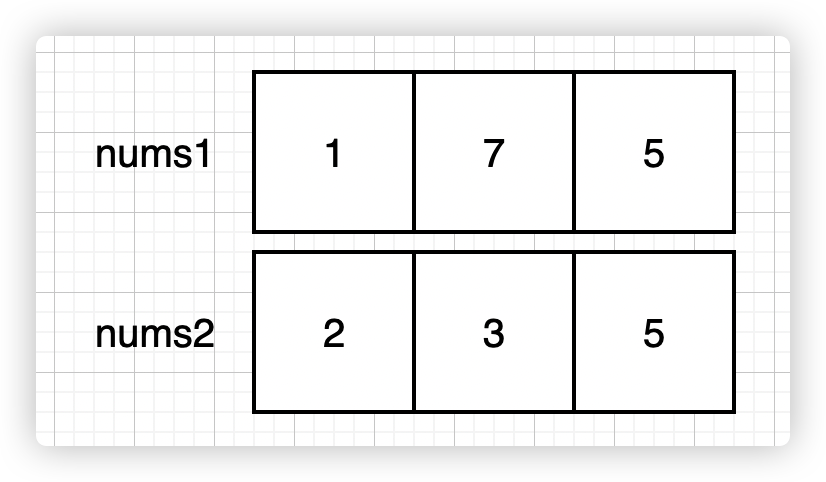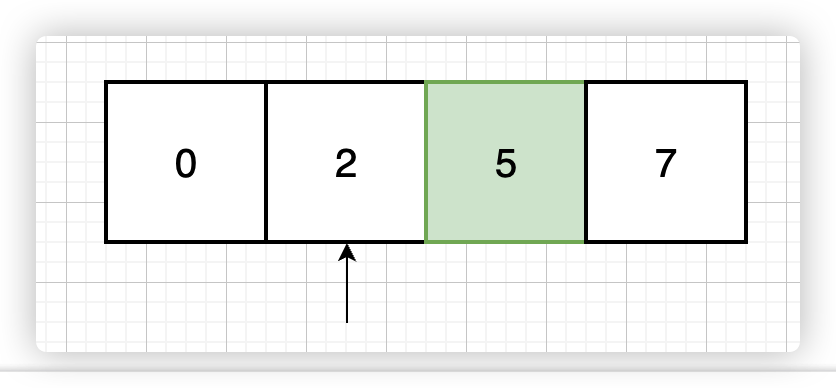## 1818. 绝对值差和

mkdir700 387 2022-09-03

# 描述

|x| 定义为：

• 如果 x >= 0 ，值为 x ，或者
• 如果 x <= 0 ，值为 -x

输入：nums1 = [1,7,5], nums2 = [2,3,5]

- 将第二个元素替换为第一个元素：[1,7,5] => [1,1,5] ，或者
- 将第二个元素替换为第三个元素：[1,7,5] => [1,5,5]



输入：nums1 = [2,4,6,8,10], nums2 = [2,4,6,8,10]



输入：nums1 = [1,10,4,4,2,7], nums2 = [9,3,5,1,7,4]



• n == nums1.length
• n == nums2.length
• 1 <= n <= 105
• 1 <= nums1[i], nums2[i] <= 105

#二分查找

# 题解

1. 选择哪个元素被替换？
2. 选择哪个元素用于替换？

1. 先找到 nums1nums2 中绝对值差最大的那一对，即找到被替换元素的位置；
2. 找到 nums1 中最小的元素，用于替换掉上一步找到的元素。

1. 先找绝对值差最大的一对，就是 (1, 9)，被替换的就是下标为 0 的这个位置；
2. 找到 nums1 中最小值 1，替换后还是 [1, 28, 21]
3. 计算 绝对值差和|1-9| + |28-21| + |21-20| = 16，而实际正确结果应该是 9

1. 选择哪个元素被替换？
2. 选择哪个元素用于替换？[1,7,5] 中选择元素进行替换，为了使整体绝对值差和小，所以我们应该尽量选择使当前差值最小的元素。

• 选择 1，新差值 new_diff = 2
• 选择 7，新差值 new_diff = 4
• 选择 5，新差值 new_diff = 2

nums1 进行排序，每次从排序后的数组中选择一个数，使其满足与 nums2[i] 差值最小的条件。

• 搜索区间: [min(nums1), max(nums2)]
• 搜索结果: 元素大小尽量靠近 nums2[i]，把 nums2[i] 看做搜索的最大值，就是找搜索区间的最右边界

1. 遍历 nums1 所有元素求绝对值差和；
2. 每遍历一个元素，就通过二分找出最适合用于替换当前元素的元素（与 nums2[i] 差值最小的元素）1. diff = 1, new_diff = 2
2. diff = 3, new_diff = 1

## 0x01 二分查找

class Solution:
def minAbsoluteSumDiff(self, nums1: List[int], nums2: List[int]) -> int:
n = len(nums1)
nums = copy.copy(nums1)
nums.sort()
# max_diff 用于保存最大(diff-new_diff)
# sum_diff 原绝对值差值和
max_diff = sum_diff = 0
for i in range(n):
a, b = nums1[i], nums2[i]
if a == b:
continue
diff = abs(a - b)
sum_diff += diff
# 以上是正常的求和操作

# 通过二分查找，找出一个较nums2[i]差值最小的元素
# 即，在 nums 升序数组中，找最右边界，相减使得差值最小
left, right = 0, n - 1
while left <= right:
mid = (left + right) >> 1
if nums[mid] <= b:
left = mid + 1
else:
right = mid - 1

# 计算新差值
new_diff = abs(nums[right] - b)
# 如果不是最后一位，则需要比较比较，看看谁的值更接近nums2[i]
if right < n - 1:
new_diff = min(new_diff, abs(nums[right+1] - b))
# 如果新的差值比之前的差值小，则暂存这个值
if new_diff < diff:
max_diff = max(max_diff, diff - new_diff)
return (sum_diff - max_diff) % (10 ** 9 + 7)